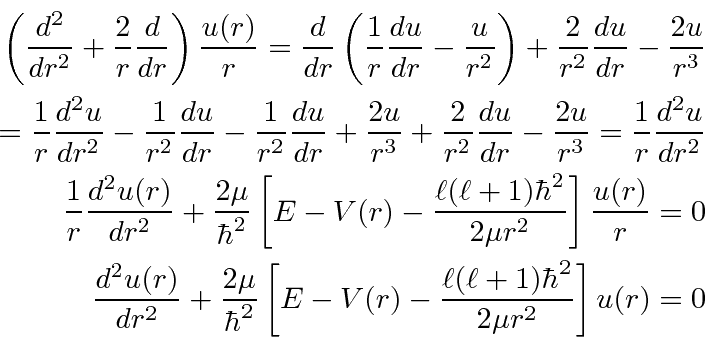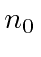## The Radial Equation for*

It is sometimes useful to useto solve a radial equation problem. We can rewrite the equation for.This now looks just like the one dimensional equation except the pseudo potential due to angular momentum has been added.

We do get the additional condition thatto keepnormalizable.

For the case of a constant potential, we defineand, and the radial equation becomes.For, its easy to see thatandare solutions. Dividing byto get, we see that these areand. The solutions can be checked for other, with some work.

Jim Branson 2013-04-22